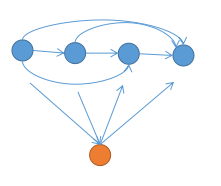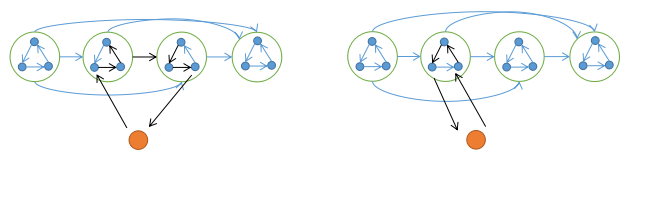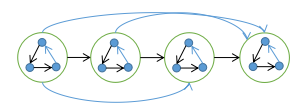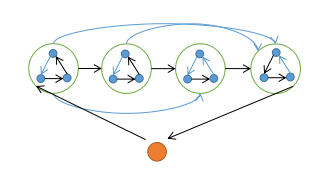# 竞赛图的一些性质

## 定理 1## 定理 2## 定理 3## 引理

n=3成立, n$\ge$4时只需证明存在大小为 $n-1$ 的就好了

(在定理3构造的哈密顿路径中, 是一段环边一条链边这样走的, 将一段环边的起点/终点删掉.)## 定理4

$s_i$为第$i$个点的出度 (竞赛中获胜的积分)
$s$排好序后, 若满足 $\sum_{i=1}^k s_i\ge \binom k 2 且 \sum s = \binom n 2$ , 定能构造出一种竞赛图, 反之不能

## 参考资料

[竞赛图判定定理]兰道定理(Landau's Theorem)介绍及其一种证明

posted @ 2018-05-15 20:55  _zwl  阅读(1725)  评论(0编辑  收藏